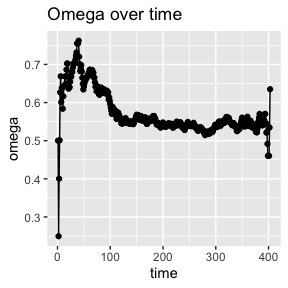# BRACE-intro

library(knitr)
library(BRACE)
#>
#> Attaching package: 'survminer'
#> The following object is masked from 'package:survival':
#>
#>     myeloma

# Introduction

The purpose of the BRACE function is to estimate and correct for certain forms of treatment selection bias arising in observational competing risks data. The function will fit Cox models for primary event(s), competing event(s), and the combined event, adjusting for confounders, following the BRACE method described in Williamson, Casey W., et al. (2022) to adjust for bias from unmeasured confounders. Results are presented in the output of $Summary table. The Cox models for primary, competing and combined endpoint, adjusting for measured confounders, are given as “Adjusted Primary Event”, “Adjusted Competing Event”, and “Adjusted Combined Event”. The proposed adjusted estimate using the BRACE method is given as “BRACE Estimate”, with a bootstrapped confidence interval. The function allows for two alternative methods for adjusting for the effects of measured confounders. One way is using multivariable Cox proportional hazards regression models. The other way is using propensity score (PS) models. For the PS method, we first estimate the propensity scores by modeling the treatment assignment with all the measured confounders through logistic regression. The propensity scores are then applied as weights to the regression models to make the patients in each group have similar distributions. Note that applying the PS method may not be sufficient to adjust for residual unmeasured confounding, which can be detected following competing event analysis. Thus, the BRACE estimate can be called even after PS adjustment. To apply BRACE method, the treatment should have an apparent benefit in reducing the hazard for competing events. Thus, if the estimate of the treatment effect on hazard for the competing event(s) is non-negative, we do not suggest using this method. The inference of the BRACE estimator is based on non-parametric bootstrap. B denotes the bootstrap sample size, with a default set to 1000. Users can reduce this number if it is computationally inefficient, but we recommend a large enough B to ensure the bootstrap consistency. The confidence interval is obtained from $$(2.5\%, 97.5\%)$$ quantile of the bootstrap samples. $BRACE HR Distribution outputs B bootstrap estimates.

Estimates of the cumulative baseline omega (relative hazard) function, omega_0, can be obtained by $Omega Estimate. This is the relative cumulative hazards for primary event(s) vs. the combined event at baseline. The approach assumes that omega_0 is approximately invariant to time (including, if applicable, any time-dependent covariates). For the convenience of assumption validity checking and sensitivity analysis, we provide a time-dependent omega_0 curve given by $omega curve.

The bias (epsilon) is given as (see Williamson, Casey W., et al (2022) for details):

$$e = (1 - \omega_0) (\Theta_2 - E(\widehat\Theta_2)) \geq (1 - \omega_0)(1 - E(\widehat\Theta_2))$$.

After BRACE adjustment, the estimate of epsilon is $$(1 - \omega_0)(1 - E(\widehat\Theta_2))$$, and can be obtained from $epsilon. # Run a toy example of brace function We generate a toy data by gendat2 function and run the brace function to get our BRACE estimator. The toy data is generated by 1. the BRACE estimator based on adjusting for the effects of measured confounders through multivariable Cox proportional hazards regression models braceoutput = brace(ftime, fstatus, covs, trt, PS=0, B=200) braceoutput$Summary
#>                          HR Estimate Lower 95% CI Upper 95% CI
#> BRACE Estimate             0.7554990    0.6766368    0.8379061
#> Adjusted Combined Event    0.5804403    0.5022396    0.6708172
#> Adjusted Primary Event     0.5430074    0.4454809    0.6618848
#> Adjusted Competing Event   0.6254957    0.5058648    0.7734179
braceoutput$Omega Curve1. the BRACE estimator based on adjusting for the effects of measured confounders through using propensity score (PS) models braceoutput = brace(ftime, fstatus, covs, trt, PS=1, B=200) braceoutput$Summary
#>                          HR Estimate Lower 95% CI Upper 95% CI
#> BRACE Estimate             0.7422218    0.6631261    0.8236102
#> Adjusted Combined Event    0.5798191    0.5053580    0.6652516
#> Adjusted Primary Event     0.5479526    0.4497555    0.6675894
#> Adjusted Competing Event   0.6162369    0.5015419    0.7571610
braceoutput\$Omega Curve